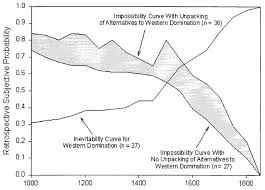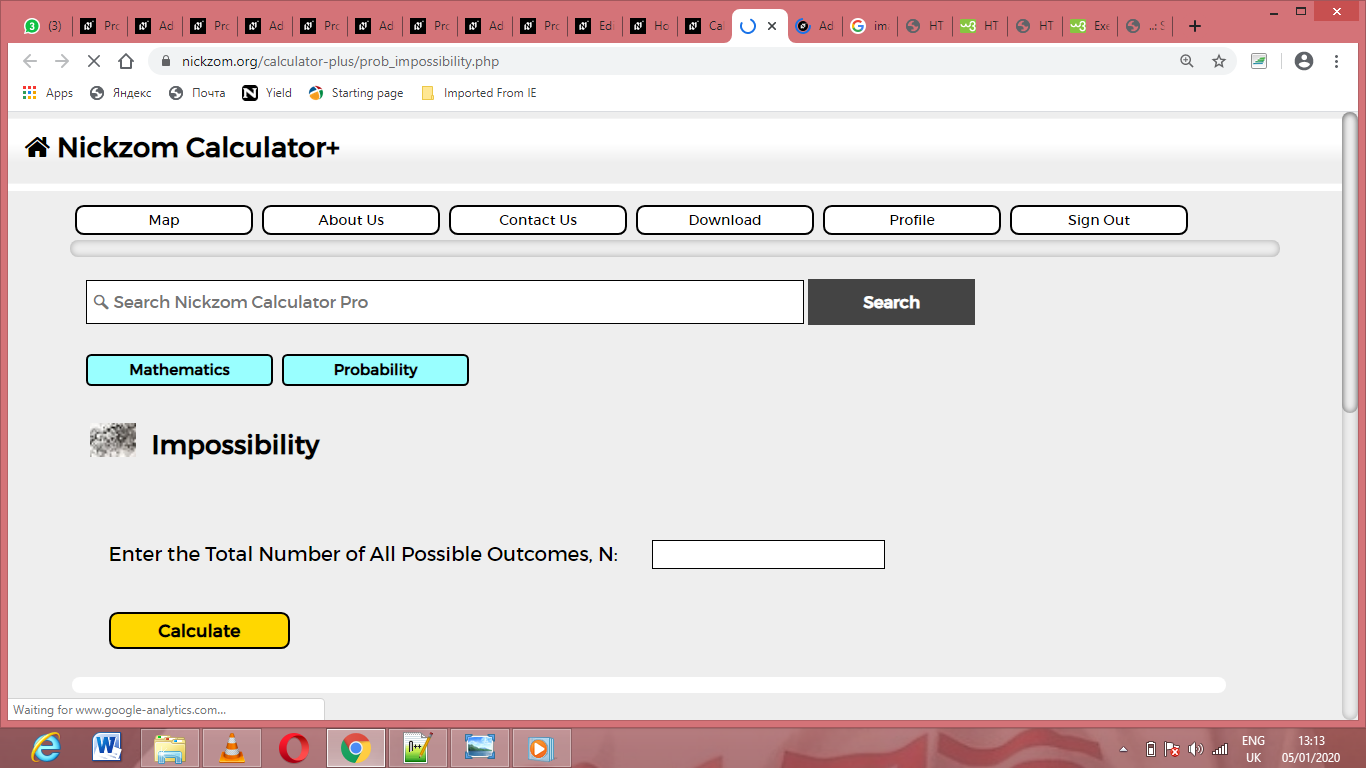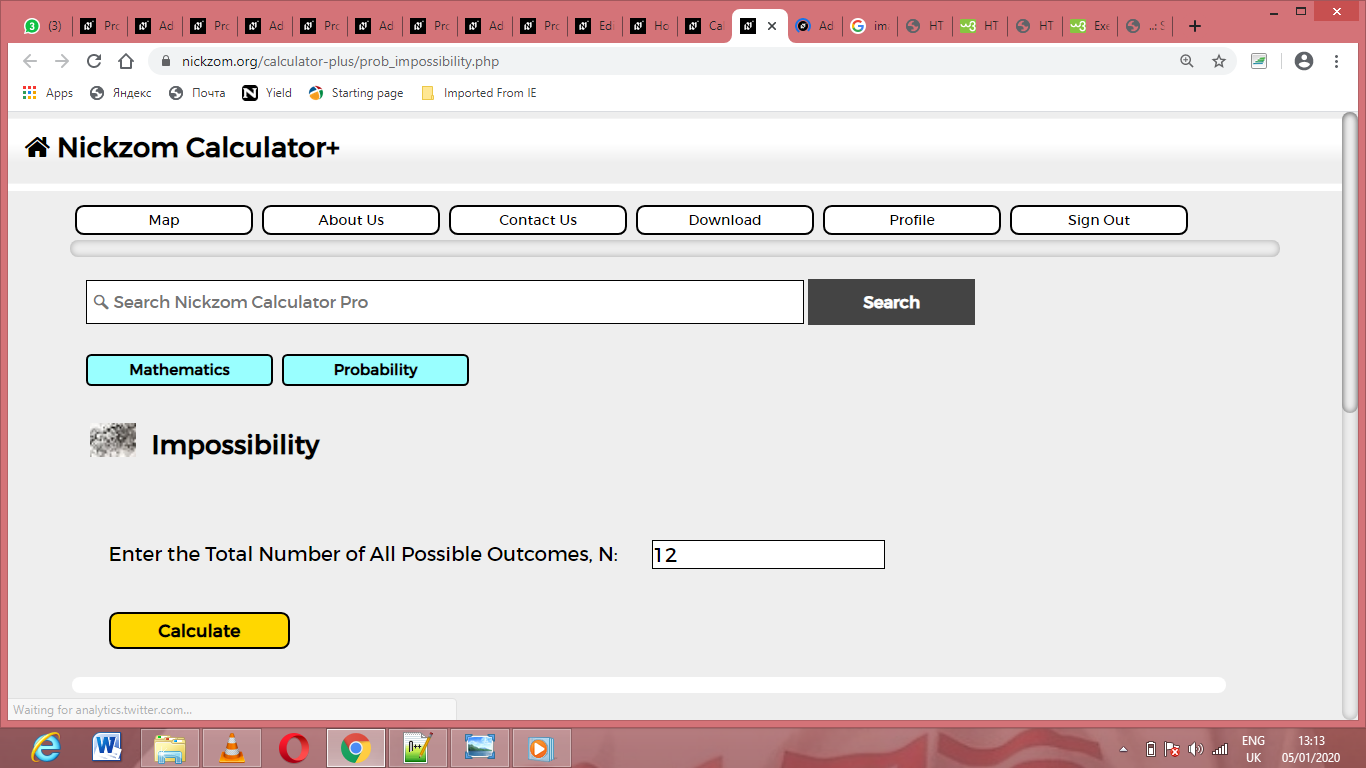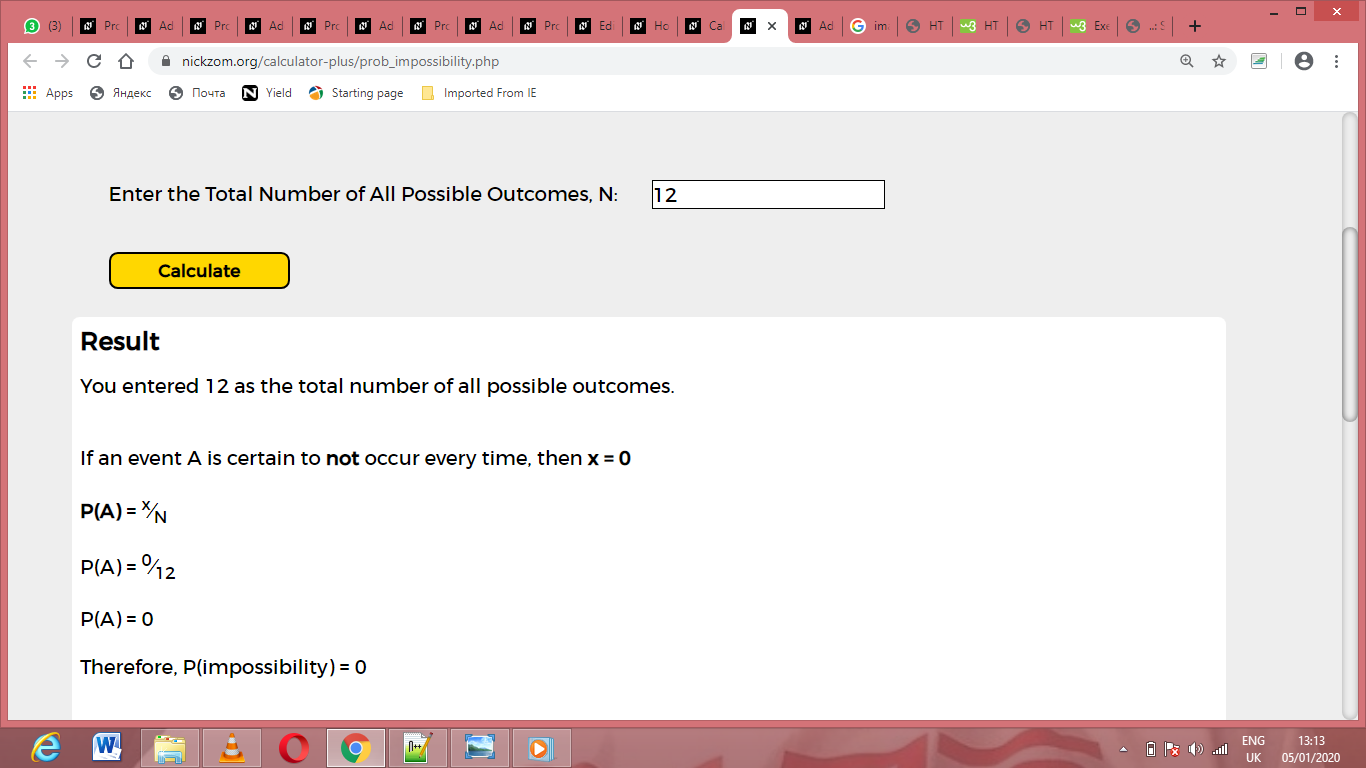# How to Calculate and Solve for Impossibility | ProbabilityThe image above represents impossibility.

To compute for impossibility, one essential parameter is needed and this parameter is Total Number of All Possible Outcomes (N).

The formula for calculating impossibility:

P(A) = x N

Where;

P(A) = Imposssibility
N = Total Number of All Possible Outcomes

Let’s solve an example;
Find the impossibility when the total number of all possible outcomes is 12.

This implies that;

N = Total Number of All Possible Outcomes = 12

P(A) = x N
P(A) = 0 12
P(A) = 0

Therefore, P(impossibility) is 0.

Nickzom Calculator – The Calculator Encyclopedia is capable of calculating the impossibility.

To get the answer and workings of the impossibility using the Nickzom Calculator – The Calculator Encyclopedia. First, you need to obtain the app.

You can get this app via any of these means:

To get access to the professional version via web, you need to register and subscribe for NGN 1,500 per annum to have utter access to all functionalities.
You can also try the demo version via https://www.nickzom.org/calculator

Apple (Paid) – https://itunes.apple.com/us/app/nickzom-calculator/id1331162702?mt=8
Once, you have obtained the calculator encyclopedia app, proceed to the Calculator Map, then click on Probability under Mathematics.Now, Click on Impossibility under ProbabilityThe screenshot below displays the page or activity to enter your value, to get the answer for the impossibility according to the respective parameter which is the Total Number of All Possible Outcomes (N).Now, enter the value appropriately and accordingly for the parameter as required by the Total Number of All Possible Outcomes (N) is 12.Finally, Click on CalculateAs you can see from the screenshot above, Nickzom Calculator– The Calculator Encyclopedia solves for the impossibility and presents the formula, workings and steps too.